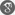##### Cite as:
Qing Zou, Some Notes on Error Analysis for Kernel Based Regularized Interpolation, Int. J. Anal. Appl., 18 (5) (2020), 689-698.

#### Abstract

Kernel based regularized interpolation is one of the most important methods for approximating functions. The theory behind the kernel based regularized interpolation is the well-known Representer Theorem, which shows the form of approximation function in the reproducing kernel Hilbert spaces. Because of the advantages of the kernel based regularized interpolation, it is widely used in many mathematical and engineering applications, for example, dimension reduction and dimension estimation. However, the performance of the approximation is not fully understood from the theoretical perspective. In other word, the error analysis for the kernel based regularized interpolation is lacking. In this paper, some error bounds in terms of the reproducing kernel Hilbert space norm and Sobolev space norm are given to understand the behavior of the approximation function.

#### References

1. M. Belkin and P. Niyogi, Semi-supervised learning on riemannian manifolds, Mach. Learn. 56 (2004), 209–239.2. M. Belkin, P. Niyogi, and V. Sindhwani, Manifold regularization: A geometric framework for learning from labeled and unlabeled examples, J. Mach. Learn. Res. 7 (2006), 2399–2434.3. R. Bhatia and C. Davis, A cauchy-schwarz inequality for operators with applications, Linear Algebra Appl. 223 (1995), 119–129.4. N. Cressie, The origins of kriging, Math. Geol. 22 (1990), 239–252.5. F. R. Deutsch, Best approximation in inner product spaces, Springer Science & Business Media, 2012.6. T. Kato, Estimation of iterated matrices, with application to the von neumann condition, Numer. Math. 2 (1960), 22–29.7. H.-J. Rong, G.-B. Huang, N. Sundararajan, and P. Saratchandran, Online sequential fuzzy extreme learning machine for function approximation and classification problems, IEEE Trans. Syst. Man Cybern. Part B (Cybernetics), 39 (2009), 1067–1072.8. B. Scholkopf, R. Herbrich, and A. J. Smola, A generalized representer theorem, in International conference on computational learning theory, Springer, 2001, 416–426.9. D. Shepard, A two-dimensional interpolation function for irregularly-spaced data, in Proceedings of the 1968 23rd ACM national conference, 1968, 517–524.10. M. Thakur, B. Samanta, and D. Chakravarty, A non-stationary geostatistical approach to multigaussian kriging for local reserve estimation, Stoch. Environ. Res. Risk Assess. 32 (2018), 2381–2404.11. J. J. Thiagarajan, P.-T. Bremer, and K. N. Ramamurthy, Multiple kernel interpolation for inverting non-linear dimensionality reduction and dimension estimation, in 2014 IEEE International Conference on Acoustics, Speech and Signal Processing (ICASSP), IEEE, 2014, 6751–6755.12. A. Wannebo, Equivalent norms for the sobolev space \$W_{0}^{m,p}(Omega)\$, Ark. Mat. 32 (1994), 245–254.